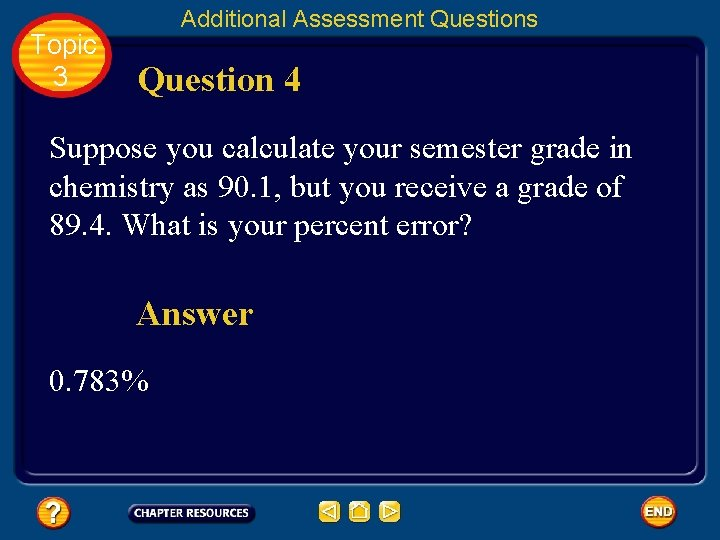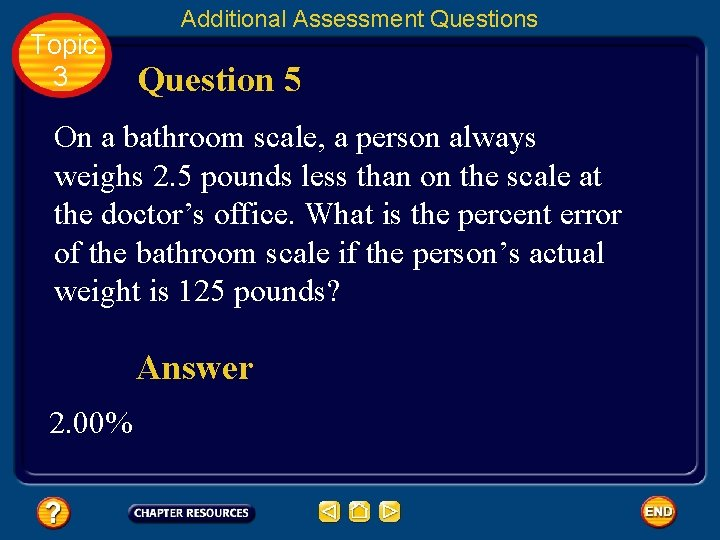• Slides: 98Topic 3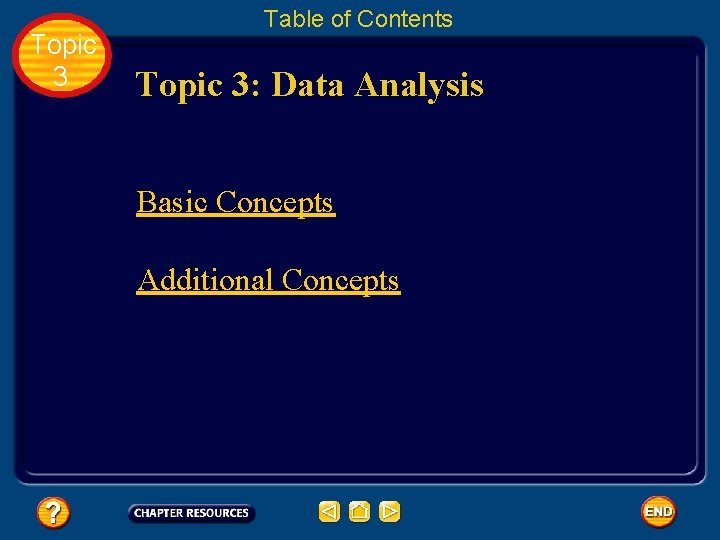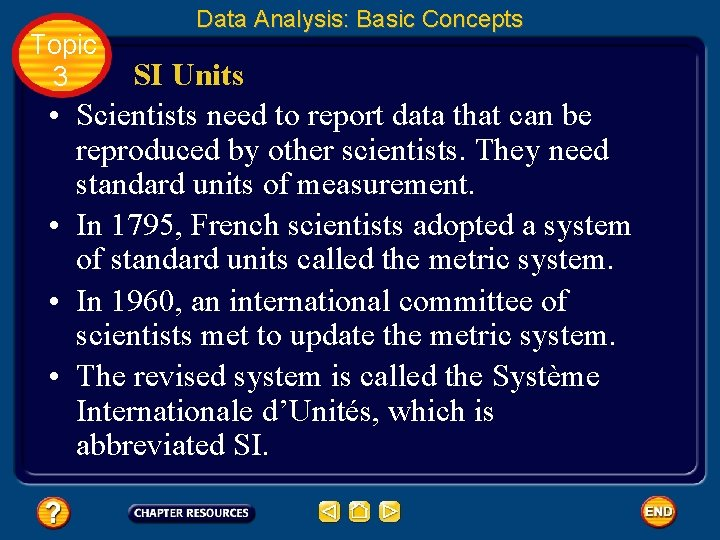Topic 3 • • Data Analysis: Basic Concepts SI Units Scientists need to report data that can be reproduced by other scientists. They need standard units of measurement. In 1795, French scientists adopted a system of standard units called the metric system. In 1960, an international committee of scientists met to update the metric system. The revised system is called the Système Internationale d’Unités, which is abbreviated SI.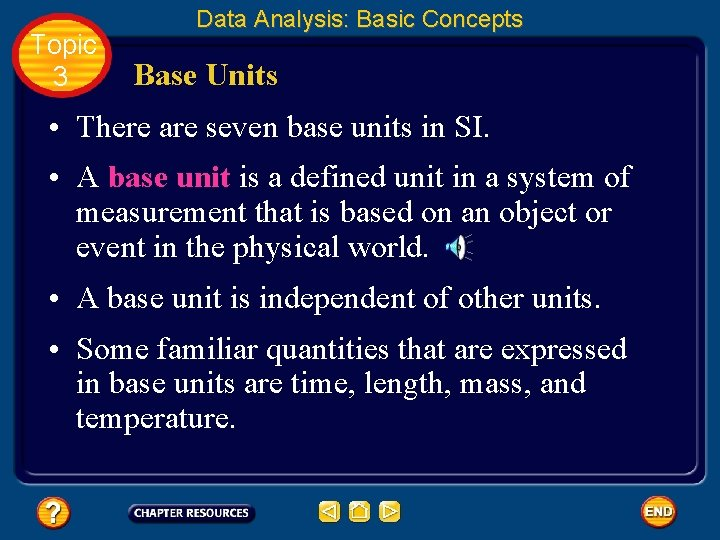Topic 3 Data Analysis: Basic Concepts Base Units • There are seven base units in SI. • A base unit is a defined unit in a system of measurement that is based on an object or event in the physical world. • A base unit is independent of other units. • Some familiar quantities that are expressed in base units are time, length, mass, and temperature.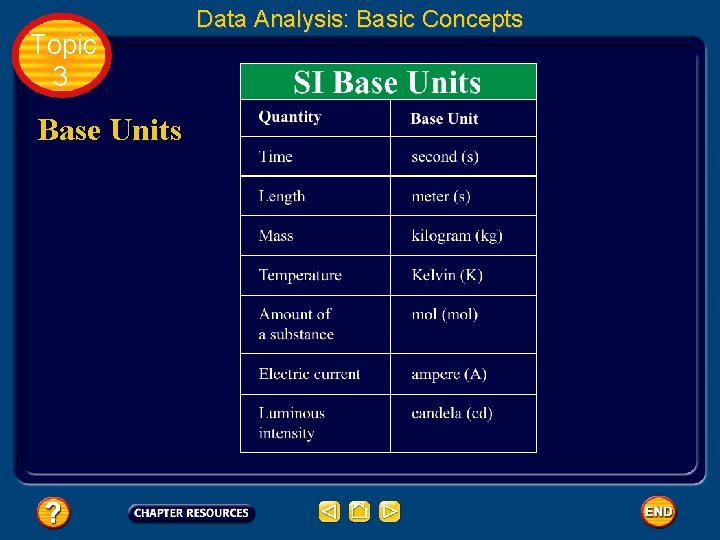Topic 3 Base Units Data Analysis: Basic ConceptsTopic 3 Data Analysis: Basic Concepts Time • The SI base unit for time is the second (s). • The frequency of microwave radiation given off by a cesium-133 atom is the physical standard used to establish the length of a second.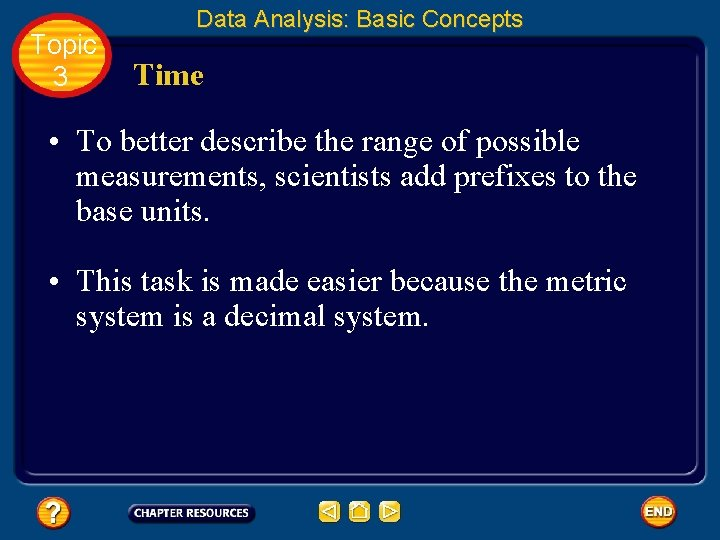Topic 3 Data Analysis: Basic Concepts Time • To better describe the range of possible measurements, scientists add prefixes to the base units. • This task is made easier because the metric system is a decimal system.Topic 3 Time Data Analysis: Basic Concepts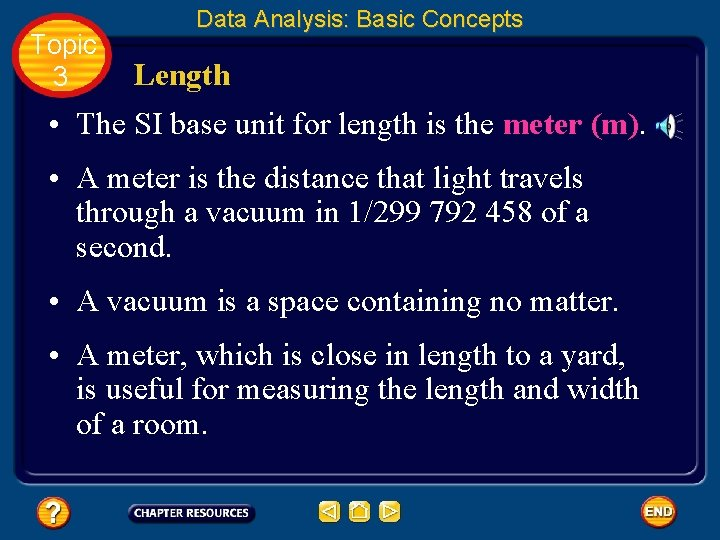Topic 3 Data Analysis: Basic Concepts Length • The SI base unit for length is the meter (m). • A meter is the distance that light travels through a vacuum in 1/299 792 458 of a second. • A vacuum is a space containing no matter. • A meter, which is close in length to a yard, is useful for measuring the length and width of a room.Topic 3 Data Analysis: Basic Concepts Mass • Recall that mass is a measure of the amount of matter. • The SI base unit for mass is the kilogram (kg). • A kilogram is about 2. 2 pounds. The kilogram is defined by a platinumiridium metal cylinder.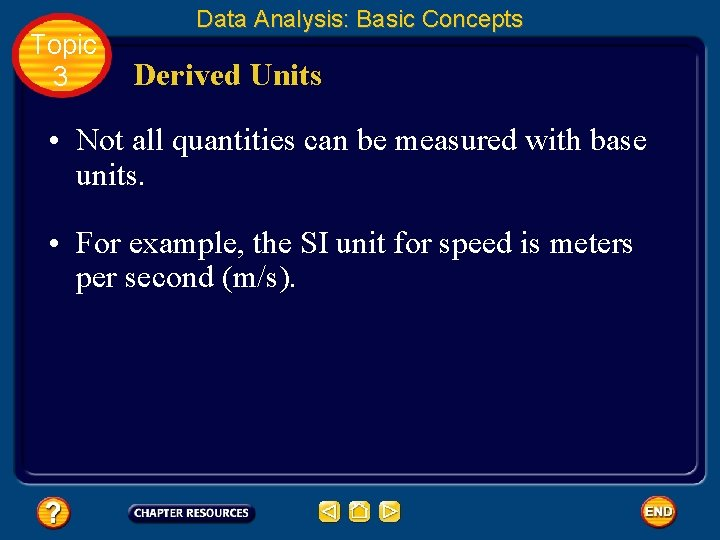Topic 3 Data Analysis: Basic Concepts Derived Units • Not all quantities can be measured with base units. • For example, the SI unit for speed is meters per second (m/s).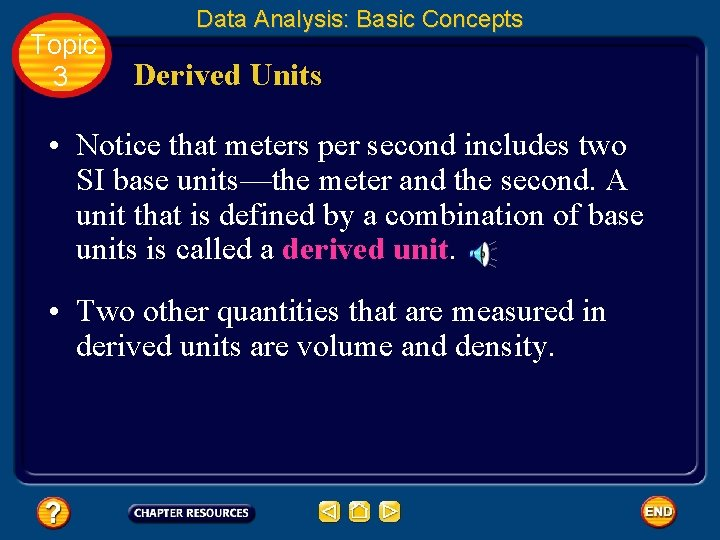Topic 3 Data Analysis: Basic Concepts Derived Units • Notice that meters per second includes two SI base units—the meter and the second. A unit that is defined by a combination of base units is called a derived unit. • Two other quantities that are measured in derived units are volume and density.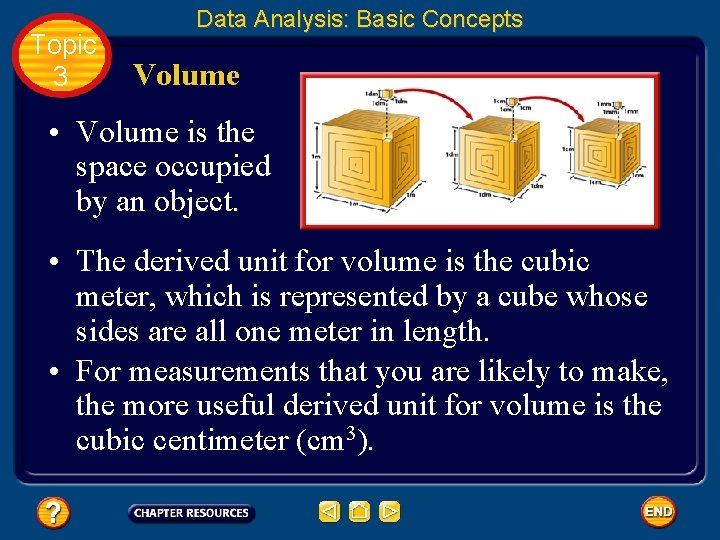Topic 3 Data Analysis: Basic Concepts Volume • Volume is the space occupied by an object. • The derived unit for volume is the cubic meter, which is represented by a cube whose sides are all one meter in length. • For measurements that you are likely to make, the more useful derived unit for volume is the cubic centimeter (cm 3).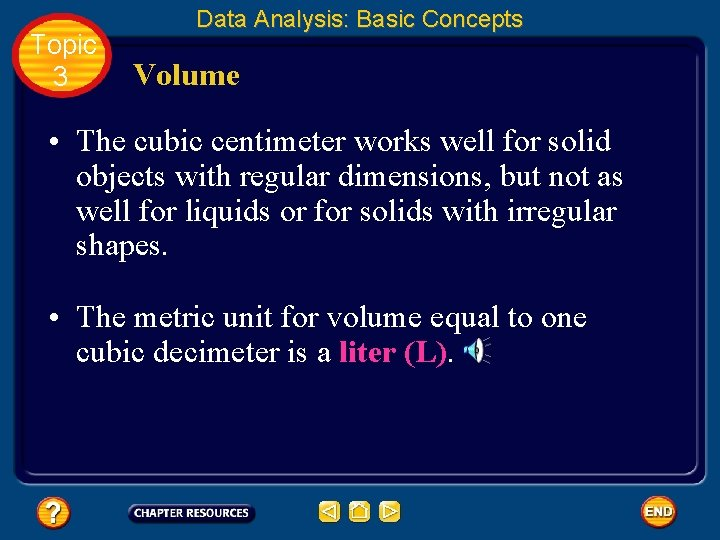Topic 3 Data Analysis: Basic Concepts Volume • The cubic centimeter works well for solid objects with regular dimensions, but not as well for liquids or for solids with irregular shapes. • The metric unit for volume equal to one cubic decimeter is a liter (L).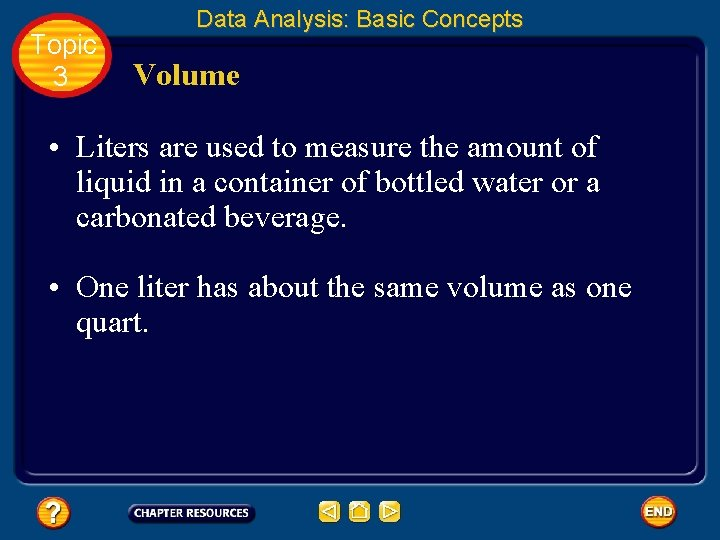Topic 3 Data Analysis: Basic Concepts Volume • Liters are used to measure the amount of liquid in a container of bottled water or a carbonated beverage. • One liter has about the same volume as one quart.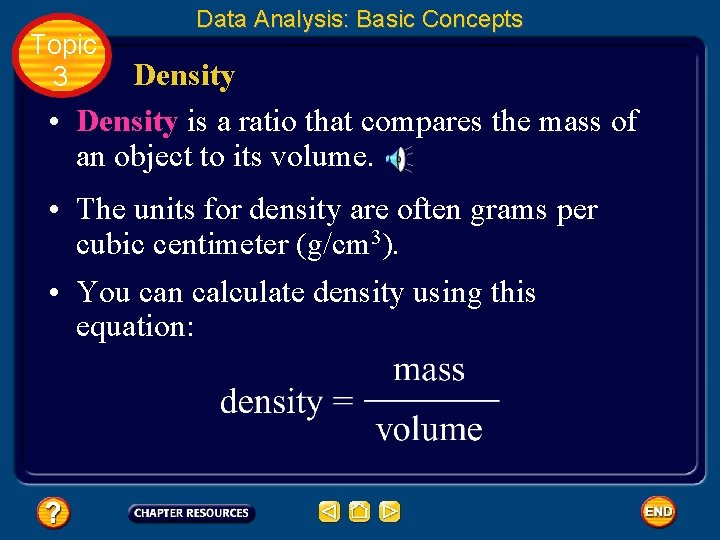Topic 3 Data Analysis: Basic Concepts Density • Density is a ratio that compares the mass of an object to its volume. • The units for density are often grams per cubic centimeter (g/cm 3). • You can calculate density using this equation: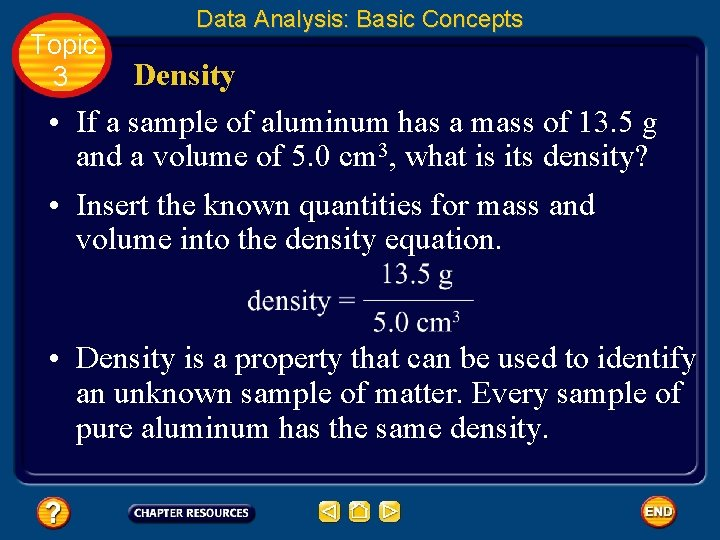Topic 3 Data Analysis: Basic Concepts Density • If a sample of aluminum has a mass of 13. 5 g and a volume of 5. 0 cm 3, what is its density? • Insert the known quantities for mass and volume into the density equation. • Density is a property that can be used to identify an unknown sample of matter. Every sample of pure aluminum has the same density.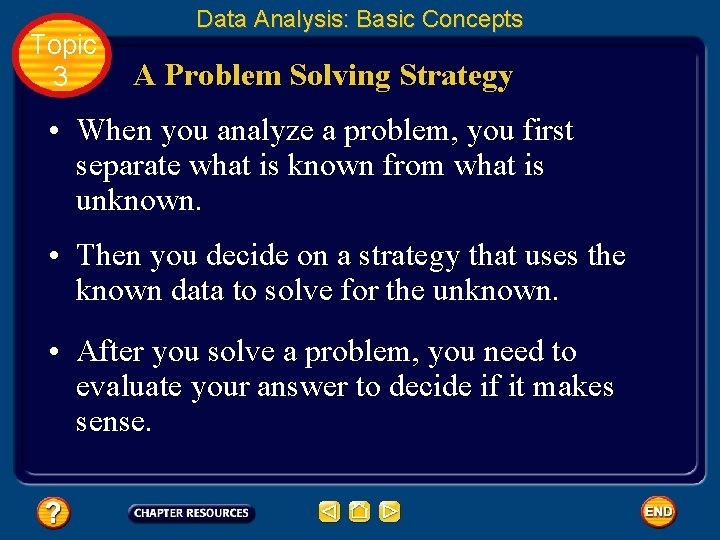Topic 3 Data Analysis: Basic Concepts A Problem Solving Strategy • When you analyze a problem, you first separate what is known from what is unknown. • Then you decide on a strategy that uses the known data to solve for the unknown. • After you solve a problem, you need to evaluate your answer to decide if it makes sense.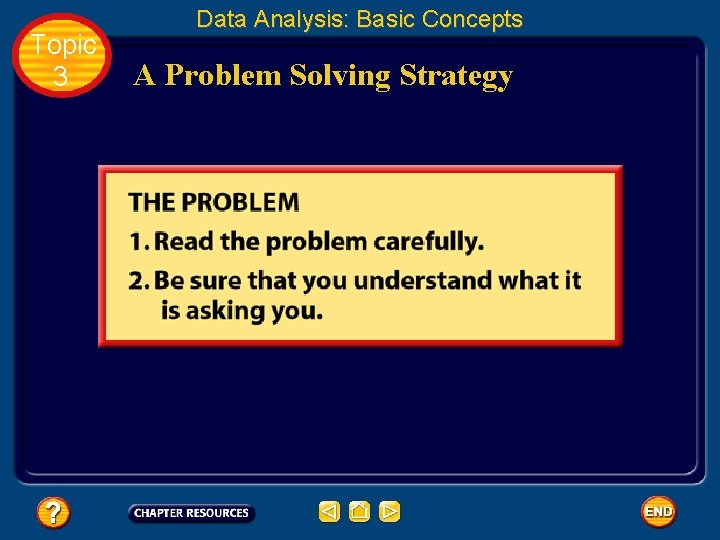Topic 3 Data Analysis: Basic Concepts A Problem Solving StrategyTopic 3 Data Analysis: Basic Concepts A Problem Solving Strategy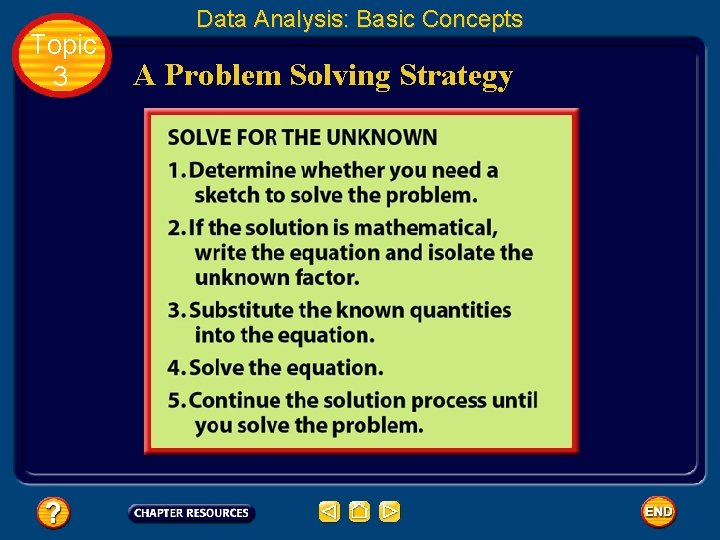Topic 3 Data Analysis: Basic Concepts A Problem Solving StrategyTopic 3 Data Analysis: Basic Concepts A Problem Solving Strategy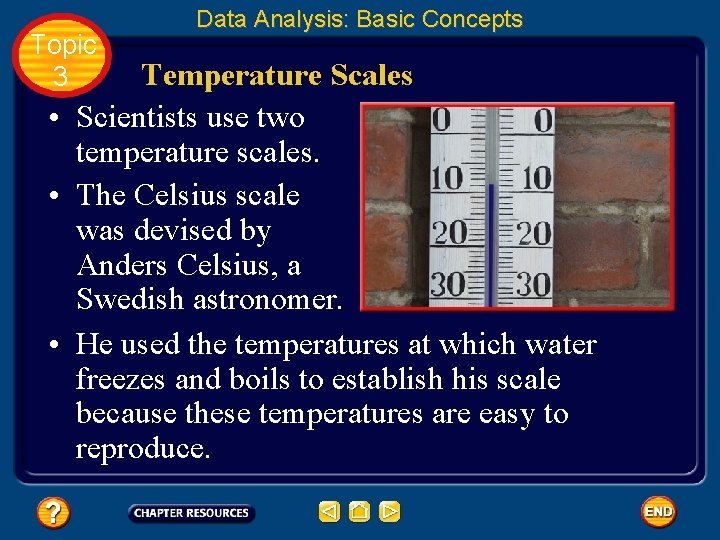Topic 3 Data Analysis: Basic Concepts Temperature Scales • Scientists use two temperature scales. • The Celsius scale was devised by Anders Celsius, a Swedish astronomer. • He used the temperatures at which water freezes and boils to establish his scale because these temperatures are easy to reproduce.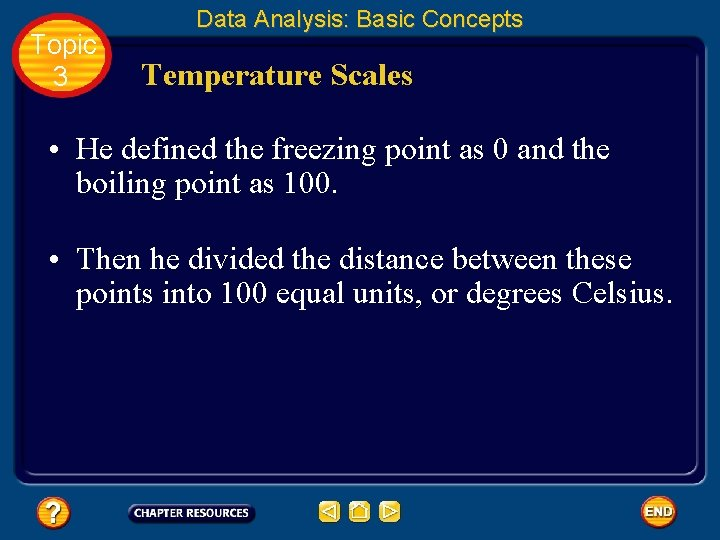Topic 3 Data Analysis: Basic Concepts Temperature Scales • He defined the freezing point as 0 and the boiling point as 100. • Then he divided the distance between these points into 100 equal units, or degrees Celsius.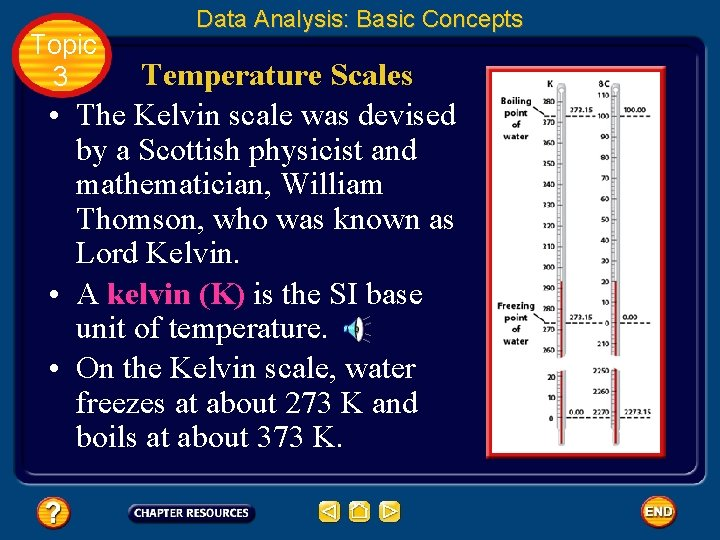Topic 3 Data Analysis: Basic Concepts Temperature Scales • The Kelvin scale was devised by a Scottish physicist and mathematician, William Thomson, who was known as Lord Kelvin. • A kelvin (K) is the SI base unit of temperature. • On the Kelvin scale, water freezes at about 273 K and boils at about 373 K.Topic 3 Data Analysis: Basic Concepts Temperature Scales • It is easy to convert from the Celsius scale to the Kelvin scale. • For example, the element mercury melts at 39 o. C and boils at 357 o. C. • To convert temperatures reported in degrees Celsius into kelvins, you just add 273.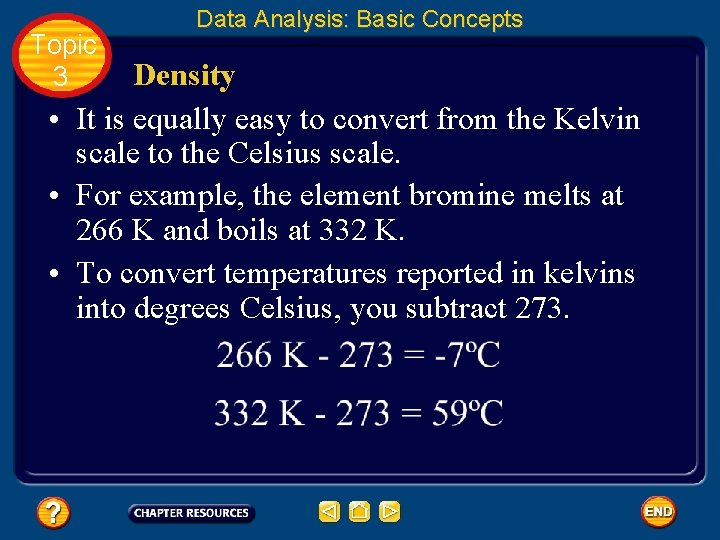Topic 3 Data Analysis: Basic Concepts Density • It is equally easy to convert from the Kelvin scale to the Celsius scale. • For example, the element bromine melts at 266 K and boils at 332 K. • To convert temperatures reported in kelvins into degrees Celsius, you subtract 273.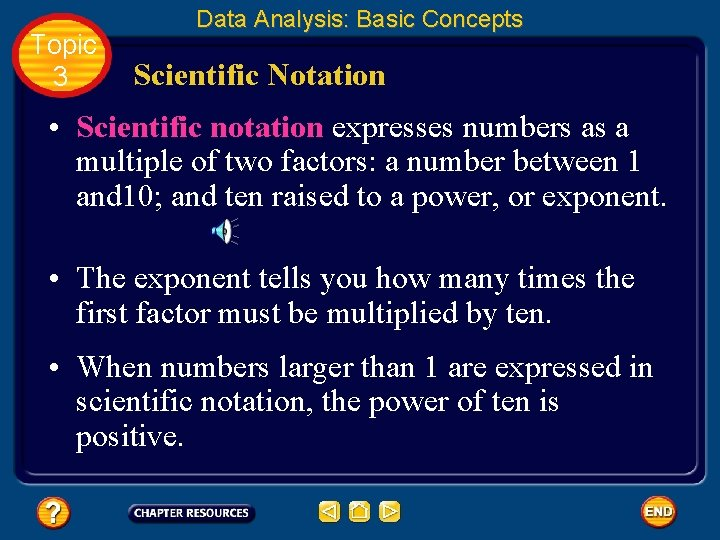Topic 3 Data Analysis: Basic Concepts Scientific Notation • Scientific notation expresses numbers as a multiple of two factors: a number between 1 and 10; and ten raised to a power, or exponent. • The exponent tells you how many times the first factor must be multiplied by ten. • When numbers larger than 1 are expressed in scientific notation, the power of ten is positive.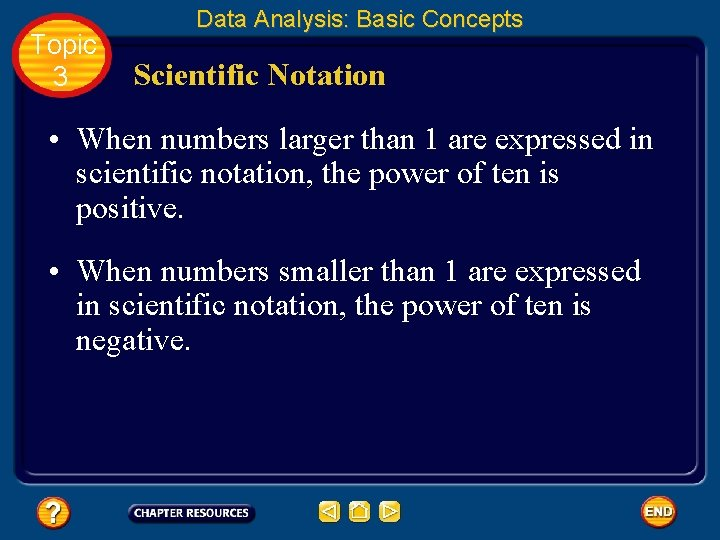Topic 3 Data Analysis: Basic Concepts Scientific Notation • When numbers larger than 1 are expressed in scientific notation, the power of ten is positive. • When numbers smaller than 1 are expressed in scientific notation, the power of ten is negative.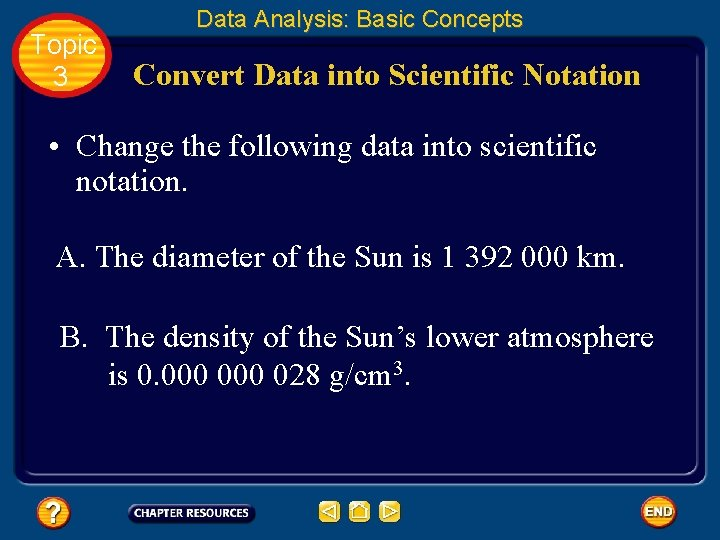Topic 3 Data Analysis: Basic Concepts Convert Data into Scientific Notation • Change the following data into scientific notation. A. The diameter of the Sun is 1 392 000 km. B. The density of the Sun’s lower atmosphere is 0. 000 028 g/cm 3.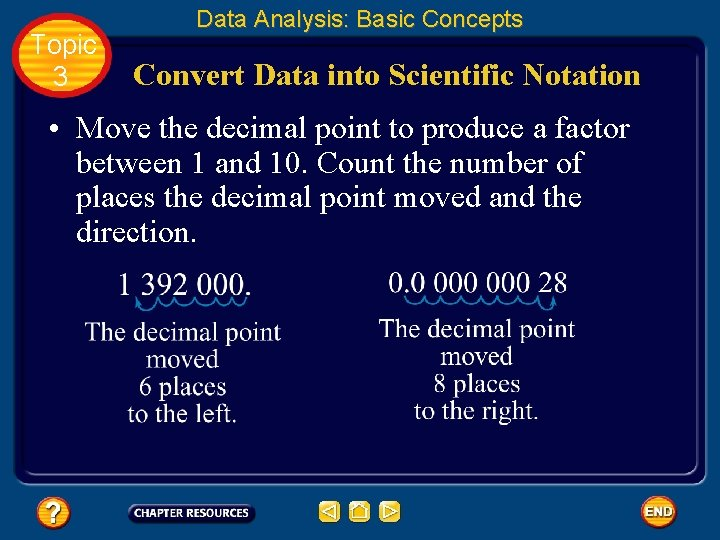Topic 3 Data Analysis: Basic Concepts Convert Data into Scientific Notation • Move the decimal point to produce a factor between 1 and 10. Count the number of places the decimal point moved and the direction.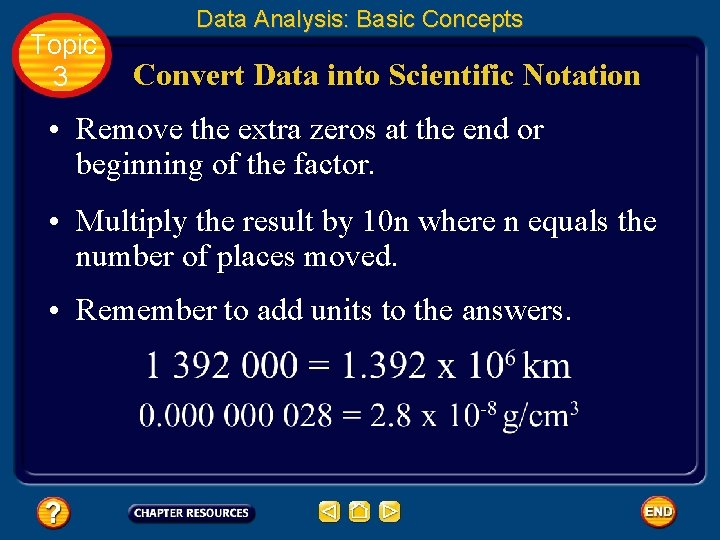Topic 3 Data Analysis: Basic Concepts Convert Data into Scientific Notation • Remove the extra zeros at the end or beginning of the factor. • Multiply the result by 10 n where n equals the number of places moved. • Remember to add units to the answers.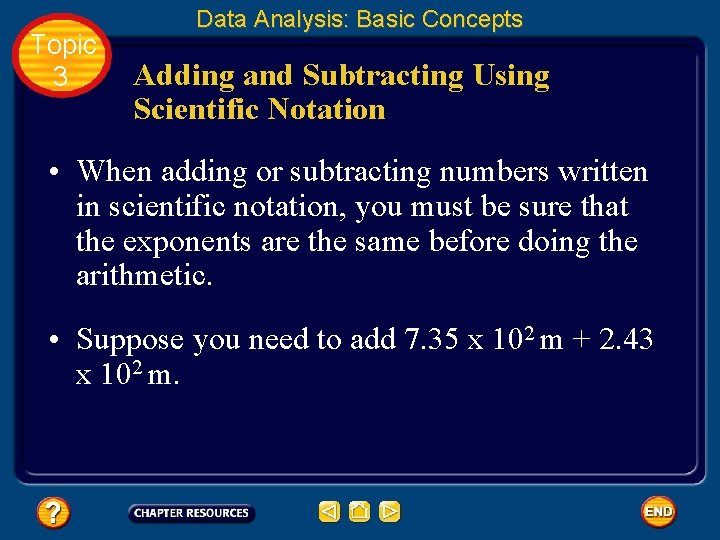Topic 3 Data Analysis: Basic Concepts Adding and Subtracting Using Scientific Notation • When adding or subtracting numbers written in scientific notation, you must be sure that the exponents are the same before doing the arithmetic. • Suppose you need to add 7. 35 x 102 m + 2. 43 x 102 m.Topic 3 Data Analysis: Basic Concepts Adding and Subtracting Using Scientific Notation • You note that the quantities are expressed to the same power of ten. You can add 7. 35 and 2. 43 to get 9. 78 x 102 m. • If the quantities are not expressed to the same power of ten, change one of the numbers to match the power of ten of the other number.Topic 3 Data Analysis: Basic Concepts Multiplying and Dividing Using Scientific Notation • Multiplying and dividing also involve two steps, but in these cases the quantities being multiplied or divided do not have to have the same exponent. • For multiplication, you multiply the first factors. Then, you add the exponents. • For division, you divide the first factors. Then, you subtract the exponent of the divisor from the exponent of the dividend.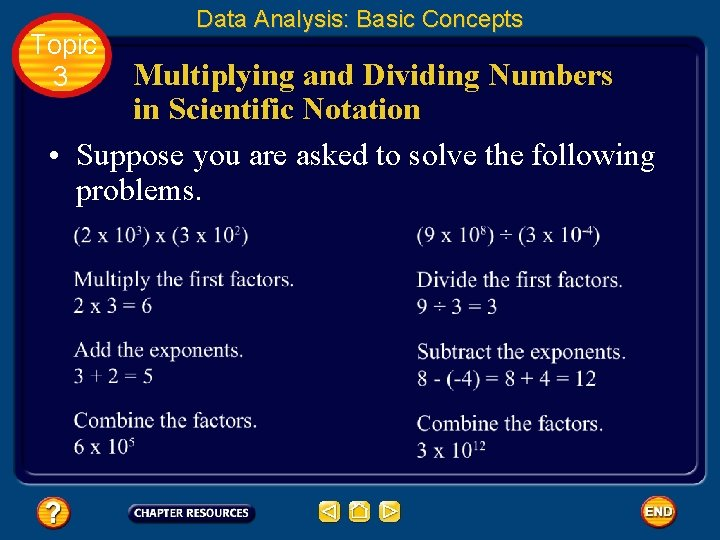Topic 3 Data Analysis: Basic Concepts Multiplying and Dividing Numbers in Scientific Notation • Suppose you are asked to solve the following problems.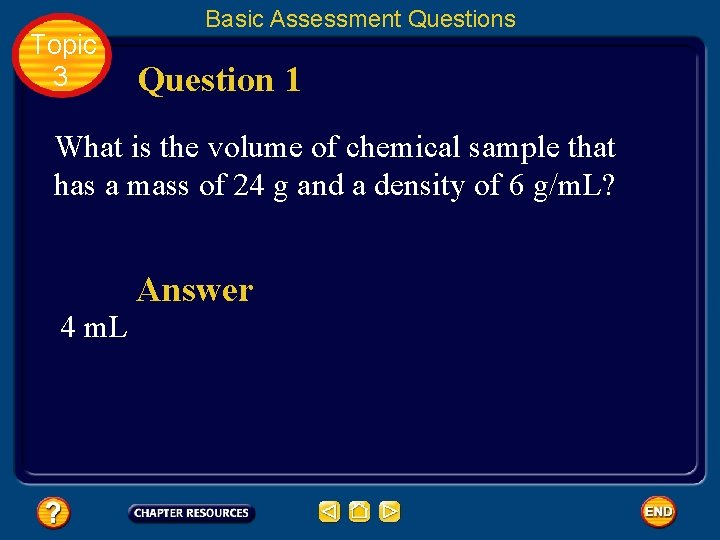Topic 3 Basic Assessment Questions Question 1 What is the volume of chemical sample that has a mass of 24 g and a density of 6 g/m. L? 4 m. L Answer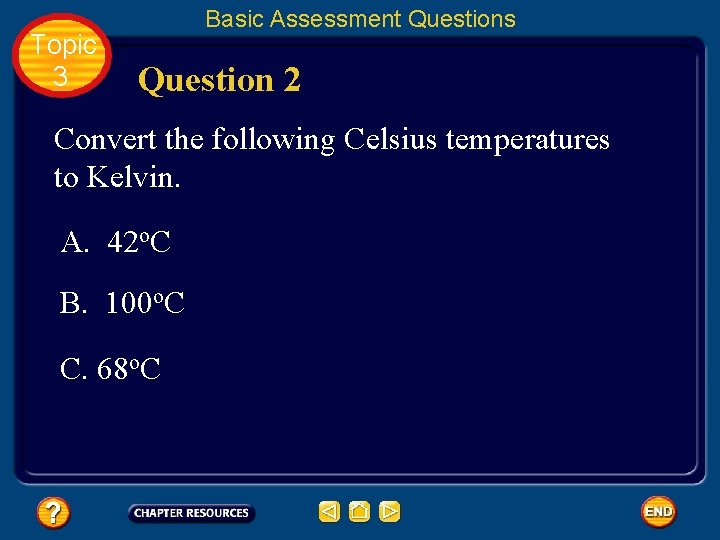Topic 3 Basic Assessment Questions Question 2 Convert the following Celsius temperatures to Kelvin. A. 42 o. C B. 100 o. C C. 68 o. C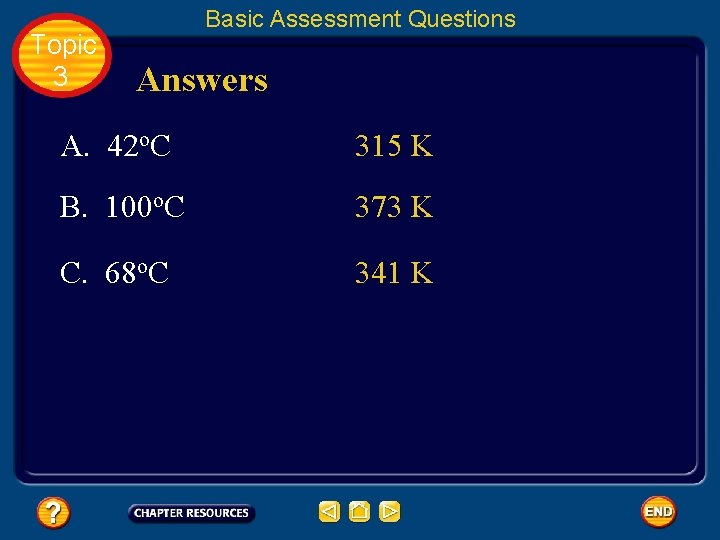Topic 3 Basic Assessment Questions Answers A. 42 o. C 315 K B. 100 o. C 373 K C. 68 o. C 341 KTopic 3 Basic Assessment Questions Question 3 Convert the following Kelvin temperatures to Celsius. A. 345 K B. 240 K C. 510 K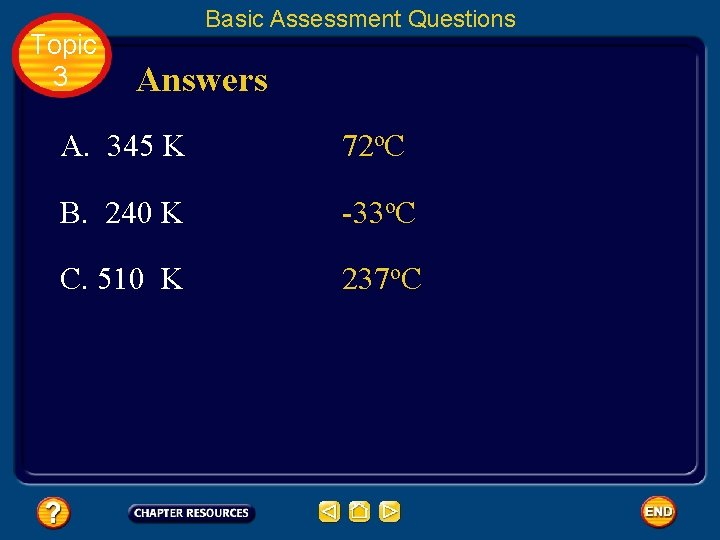Topic 3 Basic Assessment Questions Answers A. 345 K 72 o. C B. 240 K -33 o. C C. 510 K 237 o. CTopic 3 Basic Assessment Questions Question 4 Simplify the following scientific notation problems. A. B. C. D.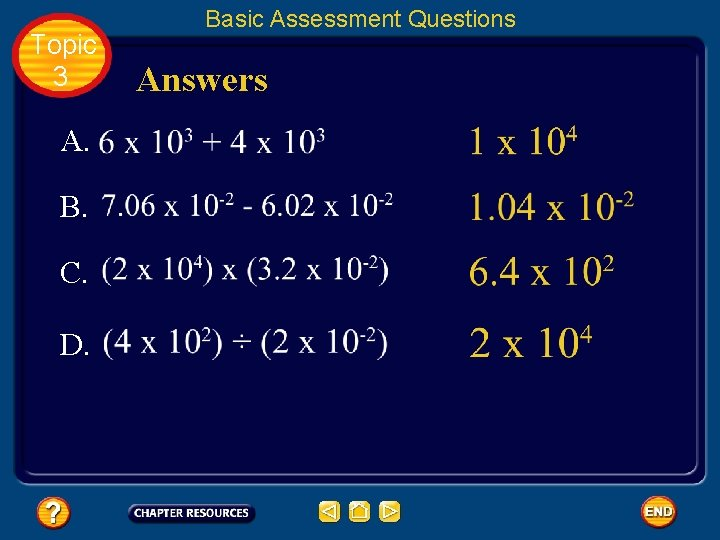Topic 3 A. B. C. D. Basic Assessment Questions Answers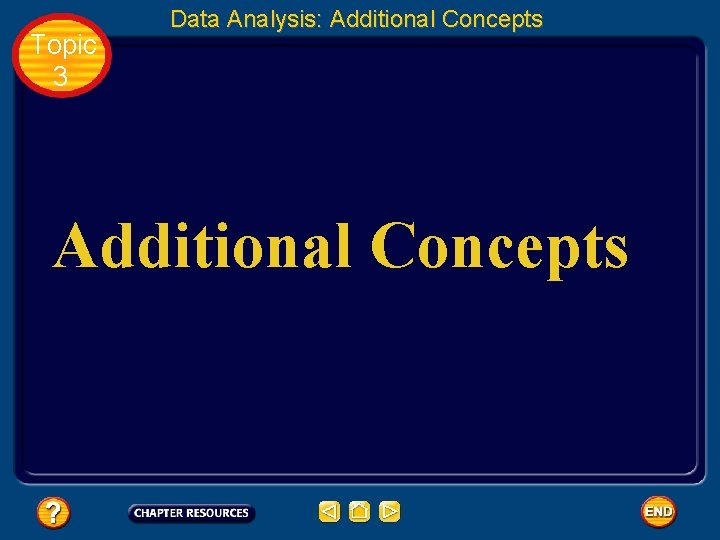Topic 3 Data Analysis: Additional Concepts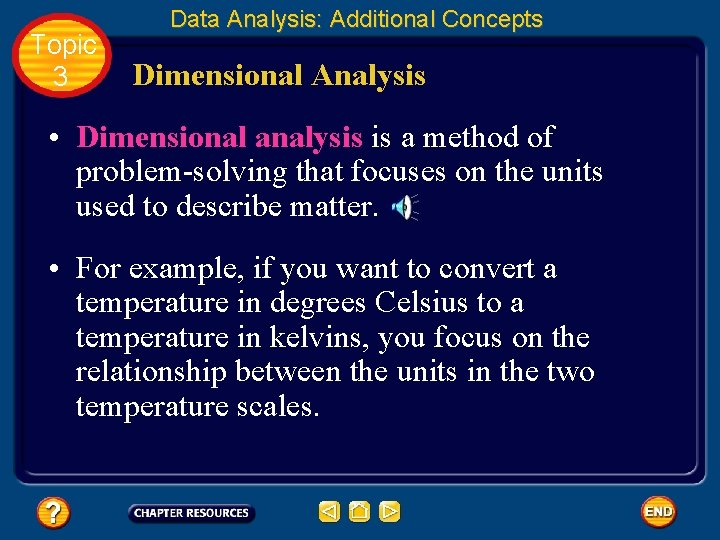Topic 3 Data Analysis: Additional Concepts Dimensional Analysis • Dimensional analysis is a method of problem-solving that focuses on the units used to describe matter. • For example, if you want to convert a temperature in degrees Celsius to a temperature in kelvins, you focus on the relationship between the units in the two temperature scales.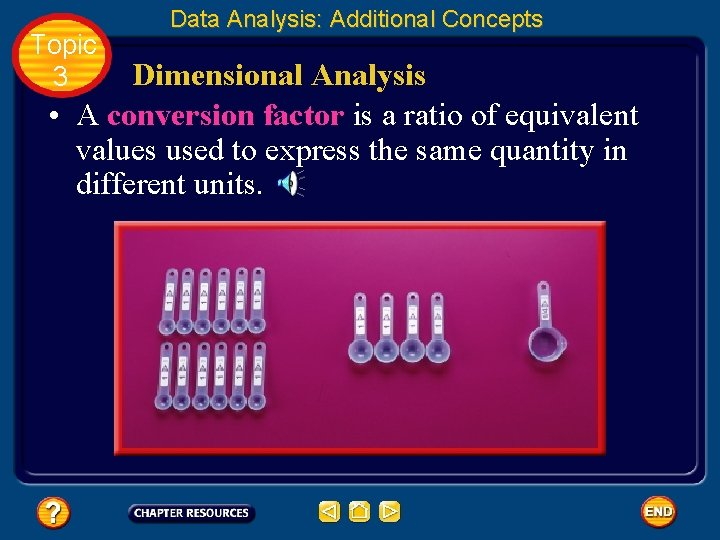Topic 3 Data Analysis: Additional Concepts Dimensional Analysis • A conversion factor is a ratio of equivalent values used to express the same quantity in different units.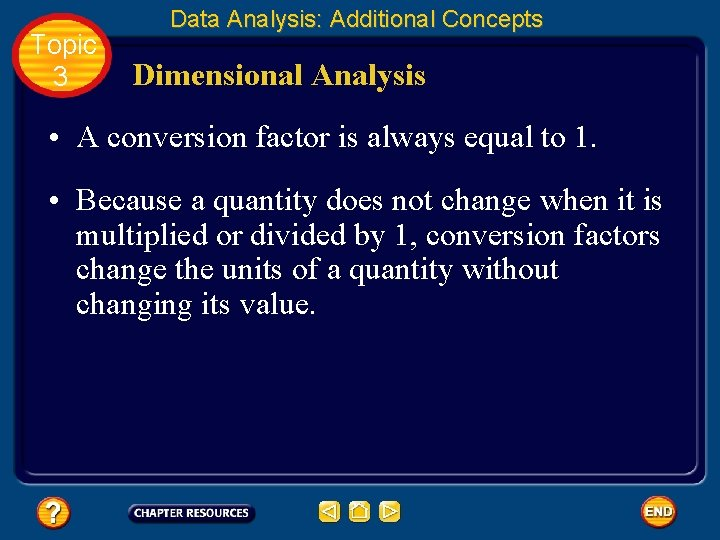Topic 3 Data Analysis: Additional Concepts Dimensional Analysis • A conversion factor is always equal to 1. • Because a quantity does not change when it is multiplied or divided by 1, conversion factors change the units of a quantity without changing its value.Topic 3 Data Analysis: Additional Concepts Dimensional Analysis • Dimensional analysis often uses conversion factors. • Suppose you want to know how many meters are in 48 km. • You need a conversion factor that relates kilometers to meters. • You know that 1 km is equal to 1000 m.Topic 3 Data Analysis: Additional Concepts Dimensional Analysis • Because you are going to multiply 48 km by the conversion factor, you want to set up the conversion factor so the kilometer units will cancel out.Topic 3 Data Analysis: Additional Concepts Using Multiple Conversion Factors • It is common in scientific problems to use dimensional analysis to convert more than one unit at a time. • For example, what is a speed of 550 meters per second in kilometers per minute?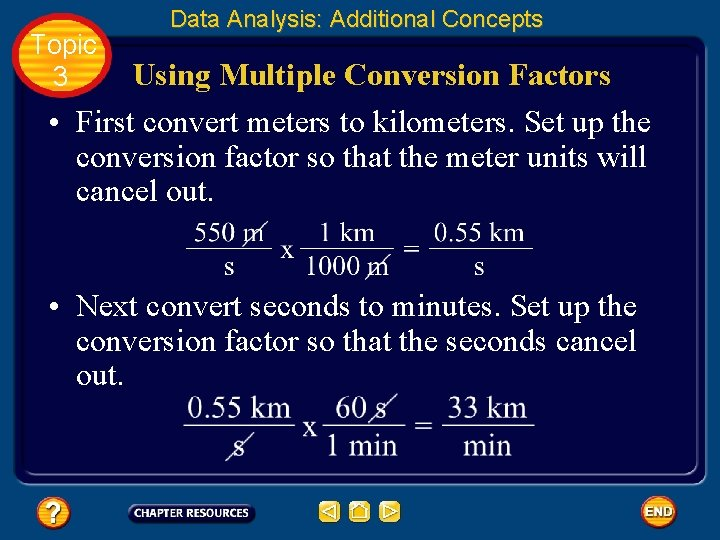Topic 3 Data Analysis: Additional Concepts Using Multiple Conversion Factors • First convert meters to kilometers. Set up the conversion factor so that the meter units will cancel out. • Next convert seconds to minutes. Set up the conversion factor so that the seconds cancel out.Topic 3 Data Analysis: Additional Concepts Accuracy and Precision • When scientists make measurements, they evaluate both the accuracy and the precision of the measurements. • Accuracy refers to how close a measured value is to an accepted value. • Precision refers to how close a series of measurements are to one another.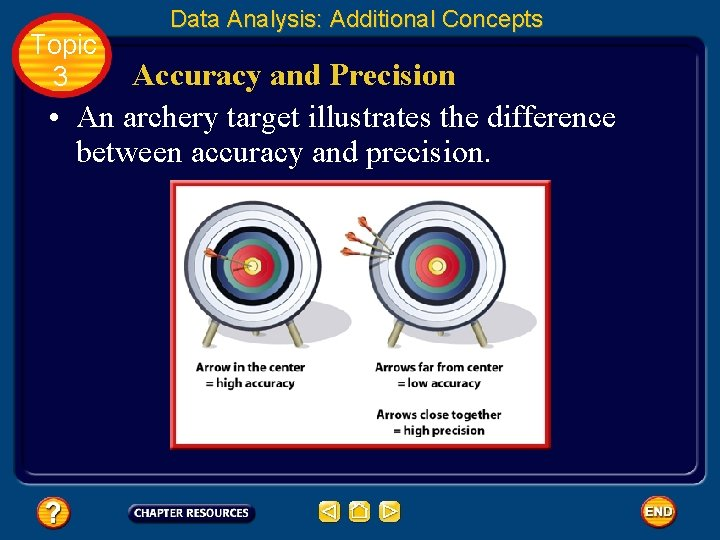Topic 3 Data Analysis: Additional Concepts Accuracy and Precision • An archery target illustrates the difference between accuracy and precision.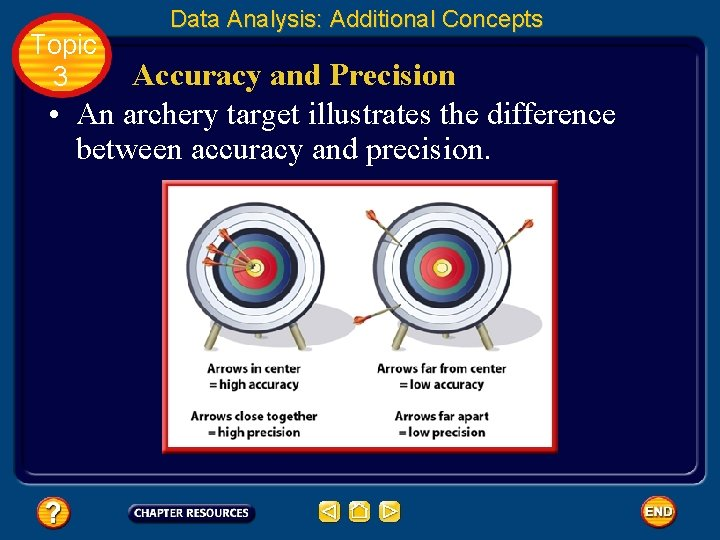Topic 3 Data Analysis: Additional Concepts Accuracy and Precision • An archery target illustrates the difference between accuracy and precision.Topic 3 Data Analysis: Additional Concepts Percent error • To evaluate the accuracy of experimental data, you can calculate the difference between an experimental value and an accepted value. • The difference is called an error.Topic 3 Data Analysis: Additional Concepts Percent error • Scientists want to know what percent of the accepted value an error represents. • Percent error is the ratio of an error to an accepted value.Topic 3 Data Analysis: Additional Concepts Percent error • For this calculation, it does not matter whether the experimental value is larger or smaller than the accepted value. Only the size of the error matters. • When you calculate percent error, you ignore plus and minus signs.Topic 3 Data Analysis: Additional Concepts Calculating Percent Error • Calculate the percent errors. Report your answers to two places after the decimal point.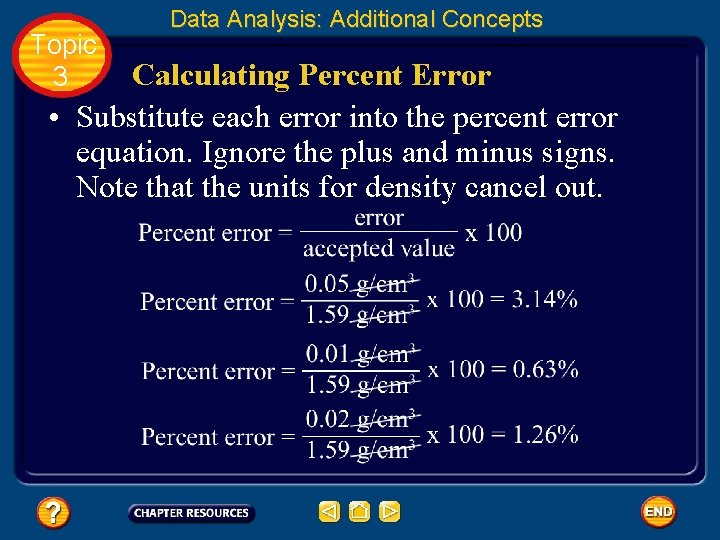Topic 3 Data Analysis: Additional Concepts Calculating Percent Error • Substitute each error into the percent error equation. Ignore the plus and minus signs. Note that the units for density cancel out.Topic 3 Data Analysis: Additional Concepts Significant Figures • Often, precision is limited by the available tools. • Scientists indicate the precision of measurements by the number of digits they report. • A value of 3. 52 g is more precise than a value of 3. 5 g.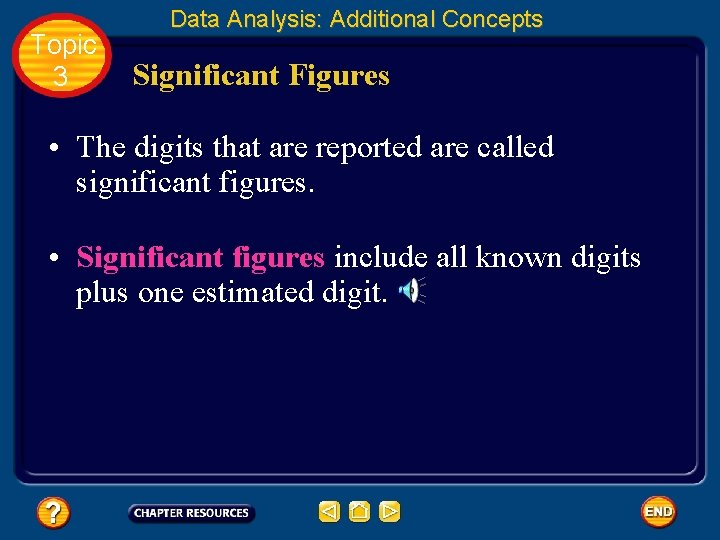Topic 3 Data Analysis: Additional Concepts Significant Figures • The digits that are reported are called significant figures. • Significant figures include all known digits plus one estimated digit.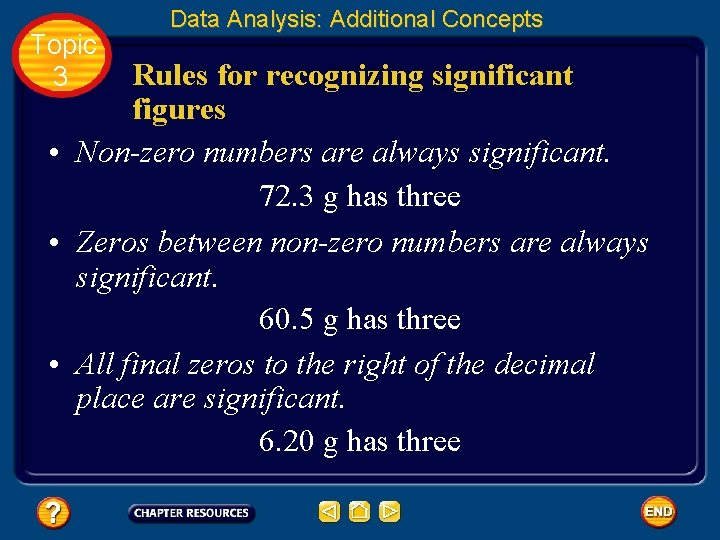Topic 3 Data Analysis: Additional Concepts Rules for recognizing significant figures • Non-zero numbers are always significant. 72. 3 g has three • Zeros between non-zero numbers are always significant. 60. 5 g has three • All final zeros to the right of the decimal place are significant. 6. 20 g has threeTopic 3 Data Analysis: Additional Concepts Rules for recognizing significant figures • Zeros that act as placeholders are not significant. Convert quantities to scientific notation to remove the placeholder zeros. 0. 0253 g and 4320 g each have three • Counting numbers and defined constants have an infinite number of significant figures. 6 molecules 60 s = 1 min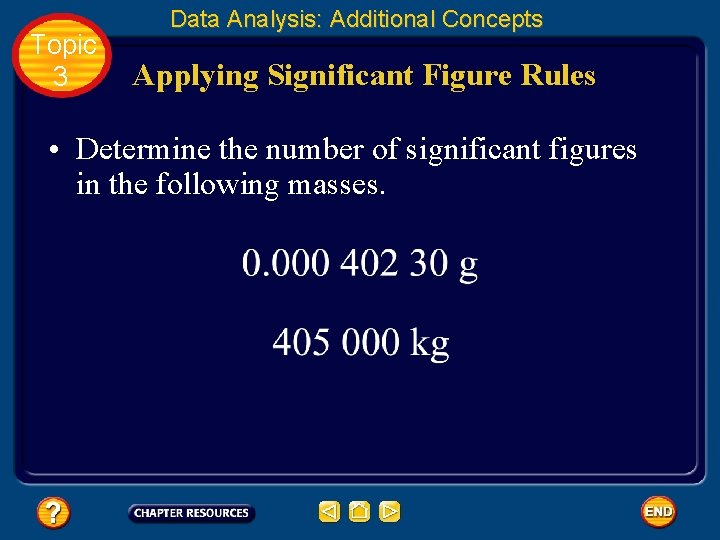Topic 3 Data Analysis: Additional Concepts Applying Significant Figure Rules • Determine the number of significant figures in the following masses.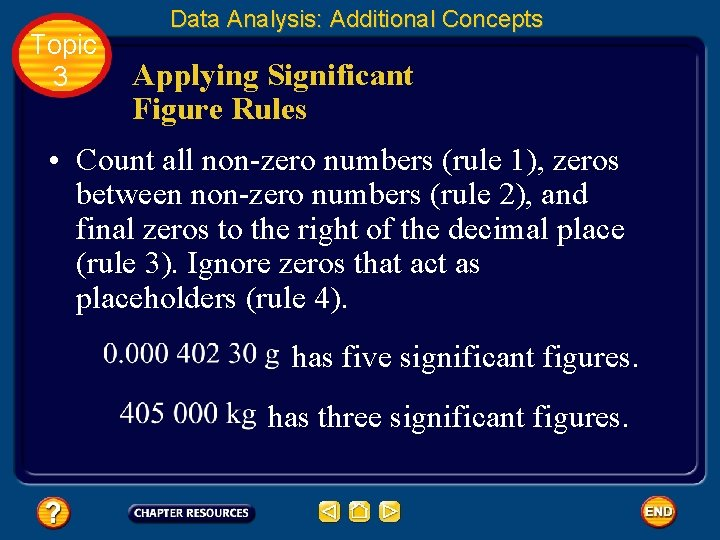Topic 3 Data Analysis: Additional Concepts Applying Significant Figure Rules • Count all non-zero numbers (rule 1), zeros between non-zero numbers (rule 2), and final zeros to the right of the decimal place (rule 3). Ignore zeros that act as placeholders (rule 4). has five significant figures. has three significant figures.Topic 3 Data Analysis: Additional Concepts Rounding Off Numbers • Answers should have no more significant figures than the data with the fewest significant figures. • In the example for each rule, there are three significant figures.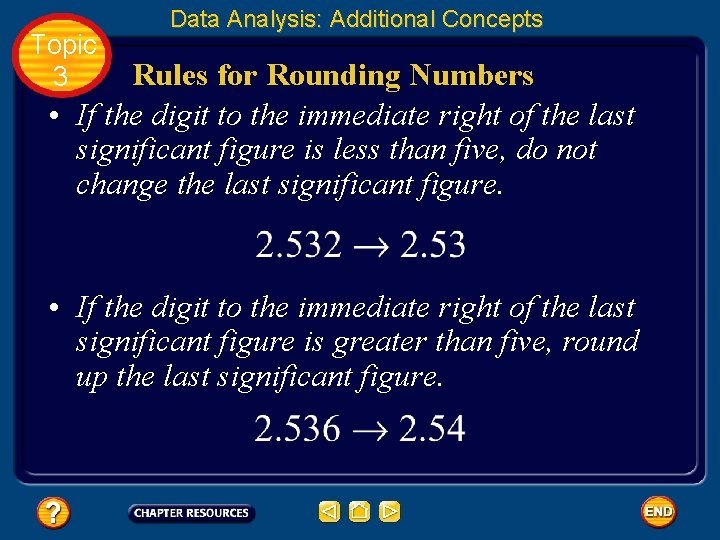Topic 3 Data Analysis: Additional Concepts Rules for Rounding Numbers • If the digit to the immediate right of the last significant figure is less than five, do not change the last significant figure. • If the digit to the immediate right of the last significant figure is greater than five, round up the last significant figure.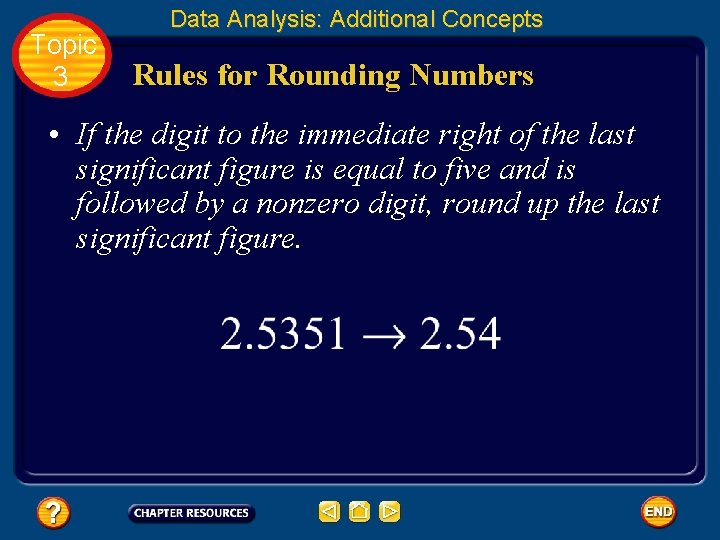Topic 3 Data Analysis: Additional Concepts Rules for Rounding Numbers • If the digit to the immediate right of the last significant figure is equal to five and is followed by a nonzero digit, round up the last significant figure.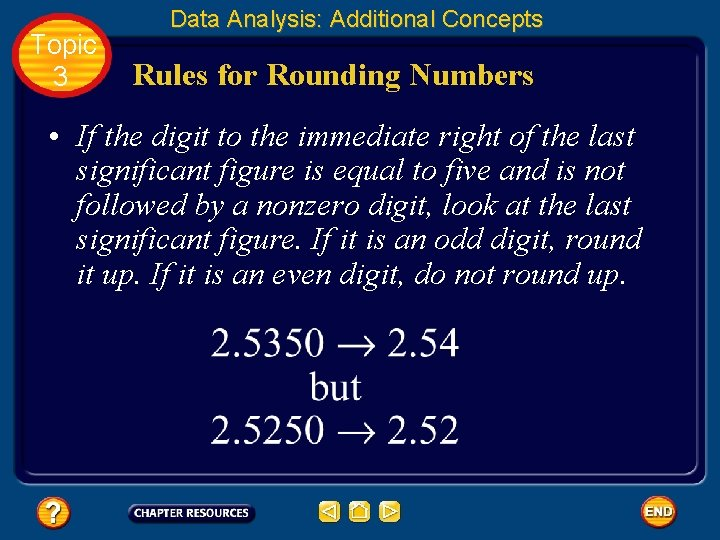Topic 3 Data Analysis: Additional Concepts Rules for Rounding Numbers • If the digit to the immediate right of the last significant figure is equal to five and is not followed by a nonzero digit, look at the last significant figure. If it is an odd digit, round it up. If it is an even digit, do not round up.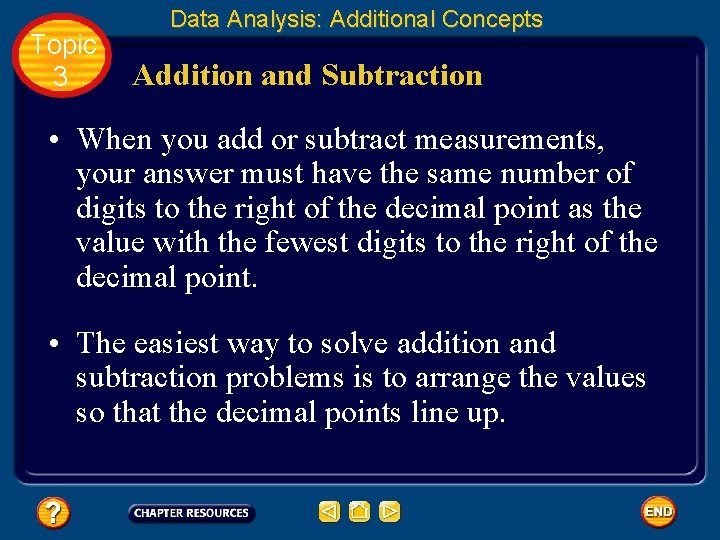Topic 3 Data Analysis: Additional Concepts Addition and Subtraction • When you add or subtract measurements, your answer must have the same number of digits to the right of the decimal point as the value with the fewest digits to the right of the decimal point. • The easiest way to solve addition and subtraction problems is to arrange the values so that the decimal points line up.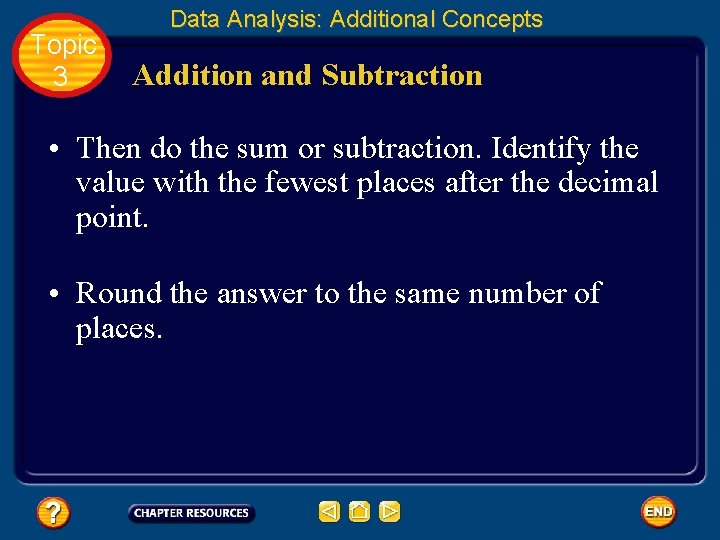Topic 3 Data Analysis: Additional Concepts Addition and Subtraction • Then do the sum or subtraction. Identify the value with the fewest places after the decimal point. • Round the answer to the same number of places.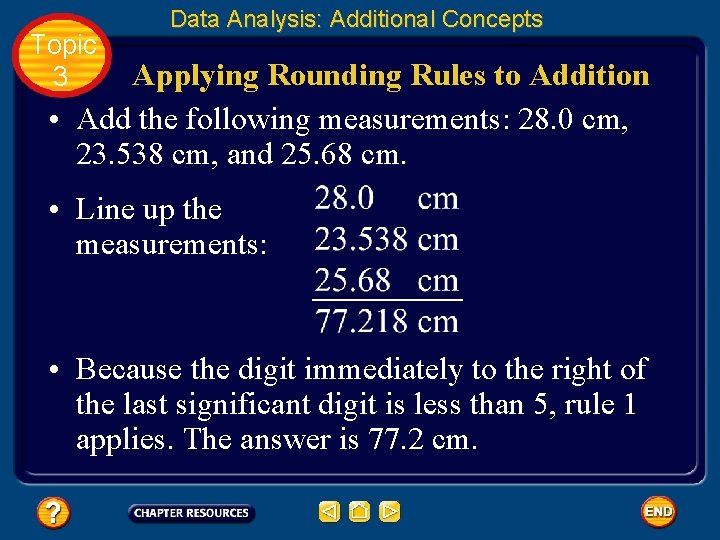Topic 3 Data Analysis: Additional Concepts Applying Rounding Rules to Addition • Add the following measurements: 28. 0 cm, 23. 538 cm, and 25. 68 cm. • Line up the measurements: • Because the digit immediately to the right of the last significant digit is less than 5, rule 1 applies. The answer is 77. 2 cm.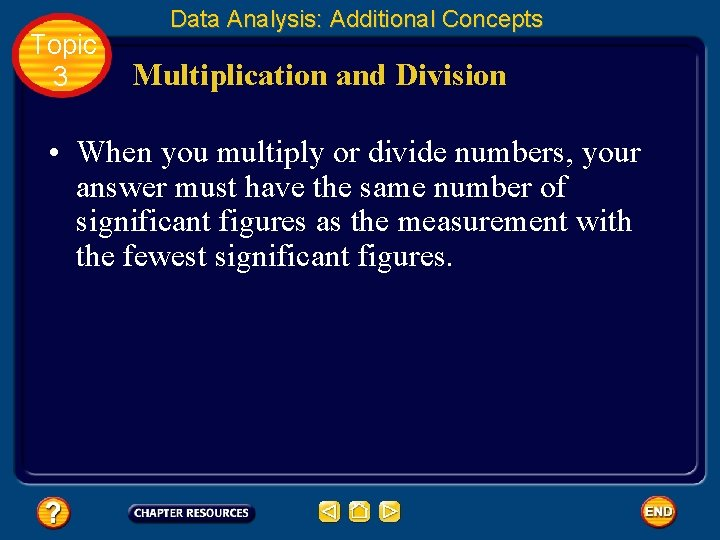Topic 3 Data Analysis: Additional Concepts Multiplication and Division • When you multiply or divide numbers, your answer must have the same number of significant figures as the measurement with the fewest significant figures.Topic 3 Data Analysis: Additional Concepts Apply Rounding Rules to Multiplication • Calculate the volume of a rectangular object with the following dimensions: length = 3. 65 cm width = 3. 20 cm height = 2. 05 cm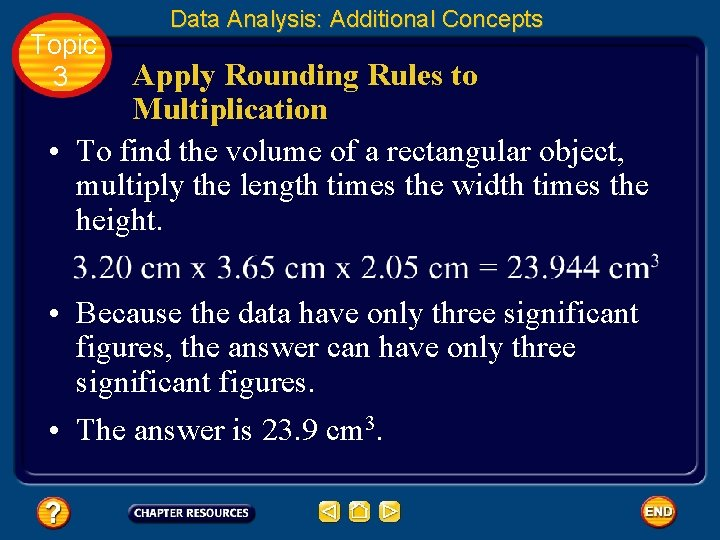Topic 3 Data Analysis: Additional Concepts Apply Rounding Rules to Multiplication • To find the volume of a rectangular object, multiply the length times the width times the height. • Because the data have only three significant figures, the answer can have only three significant figures. • The answer is 23. 9 cm 3.Topic 3 Data Analysis: Additional Concepts Graphing • Using data to create a graph can help to reveal a pattern if one exists. • A graph is a visual display of data.Topic 3 Data Analysis: Additional Concepts Circle graphs • A circle graph is sometimes called a pie chart because it is divided into wedges like a pie or pizza. • A circle graph is useful for showing parts of a fixed whole. • The parts are usually labeled as percents with the circle as a whole representing 100%.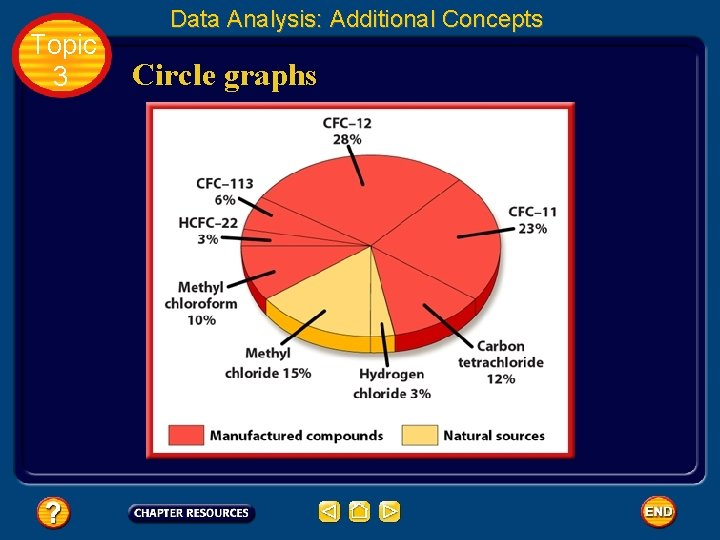Topic 3 Data Analysis: Additional Concepts Circle graphs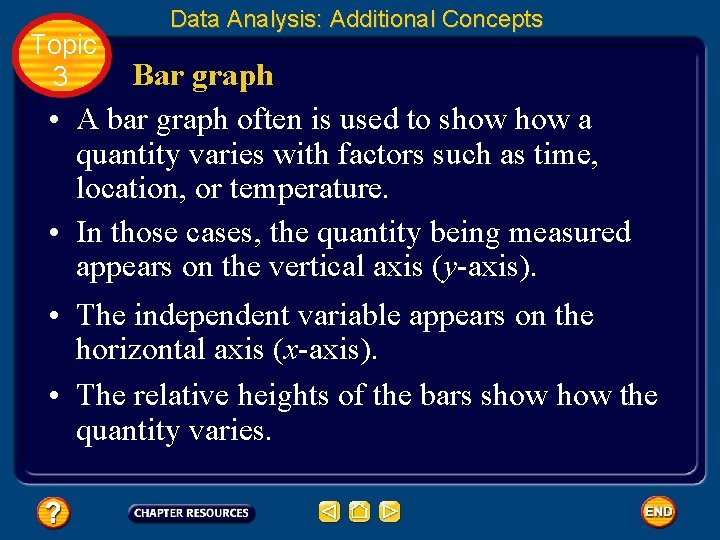Topic 3 Data Analysis: Additional Concepts Bar graph • A bar graph often is used to show a quantity varies with factors such as time, location, or temperature. • In those cases, the quantity being measured appears on the vertical axis (y-axis). • The independent variable appears on the horizontal axis (x-axis). • The relative heights of the bars show the quantity varies.Topic 3 Data Analysis: Additional Concepts Bar graph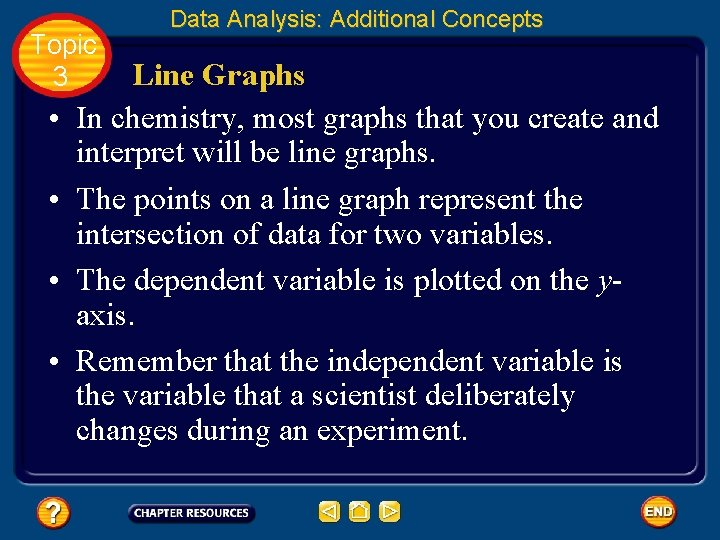Topic 3 • • Data Analysis: Additional Concepts Line Graphs In chemistry, most graphs that you create and interpret will be line graphs. The points on a line graph represent the intersection of data for two variables. The dependent variable is plotted on the yaxis. Remember that the independent variable is the variable that a scientist deliberately changes during an experiment.Topic 3 Data Analysis: Additional Concepts Line GraphsTopic 3 Data Analysis: Additional Concepts Line Graphs • Sometimes points are scattered, the line cannot pass through all the data points. • The line must be drawn so that about as many points fall above the line as fall below it. • This line is called a best fit line.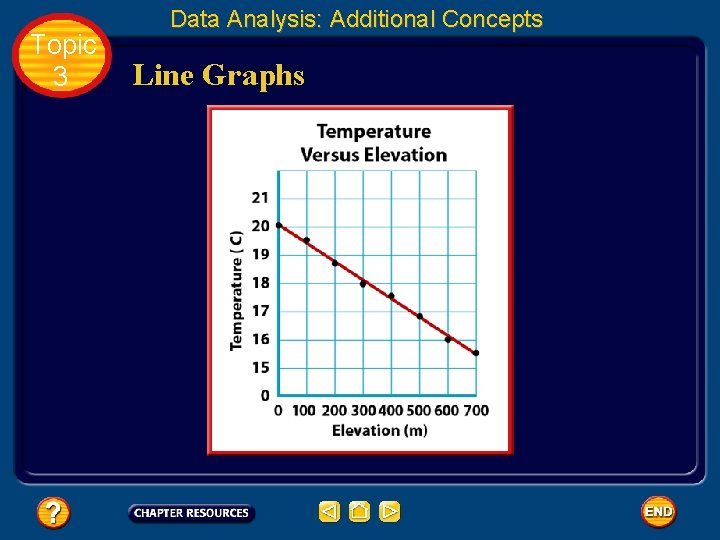Topic 3 Data Analysis: Additional Concepts Line Graphs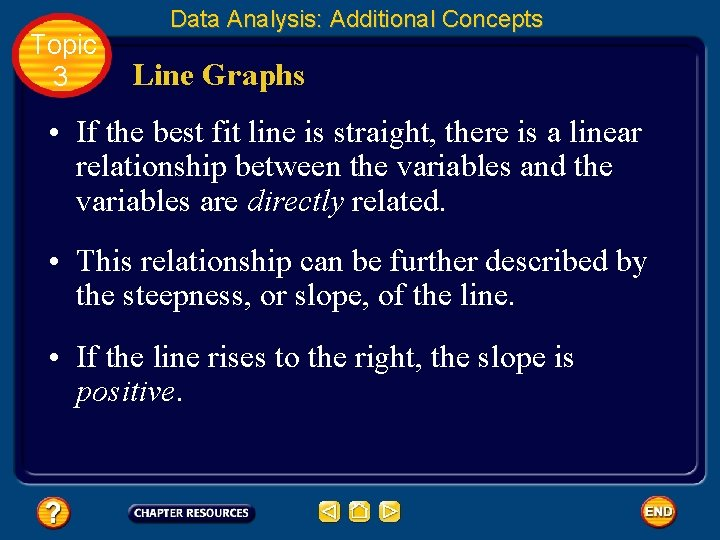Topic 3 Data Analysis: Additional Concepts Line Graphs • If the best fit line is straight, there is a linear relationship between the variables and the variables are directly related. • This relationship can be further described by the steepness, or slope, of the line. • If the line rises to the right, the slope is positive.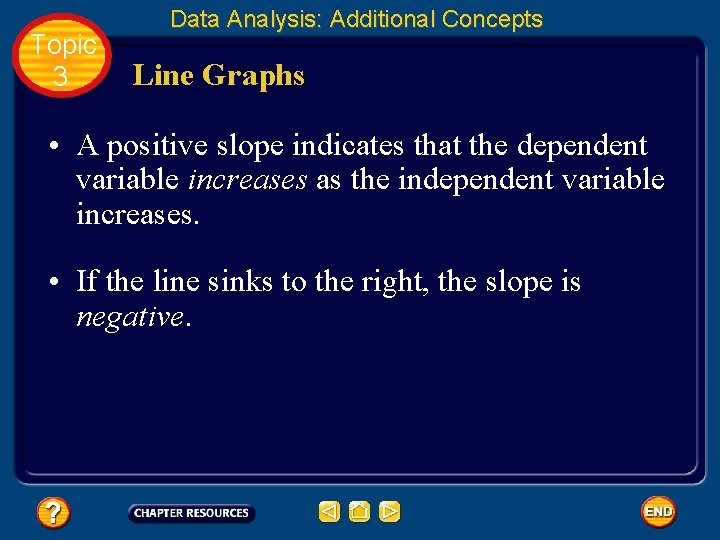Topic 3 Data Analysis: Additional Concepts Line Graphs • A positive slope indicates that the dependent variable increases as the independent variable increases. • If the line sinks to the right, the slope is negative.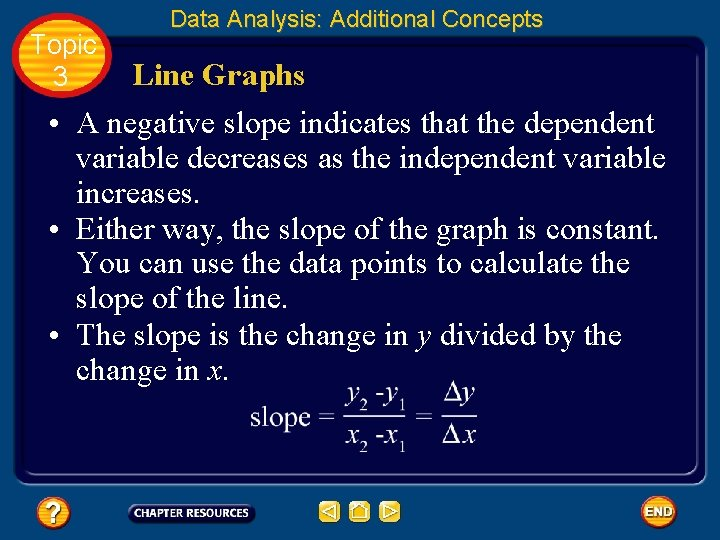Topic 3 Data Analysis: Additional Concepts Line Graphs • A negative slope indicates that the dependent variable decreases as the independent variable increases. • Either way, the slope of the graph is constant. You can use the data points to calculate the slope of the line. • The slope is the change in y divided by the change in x.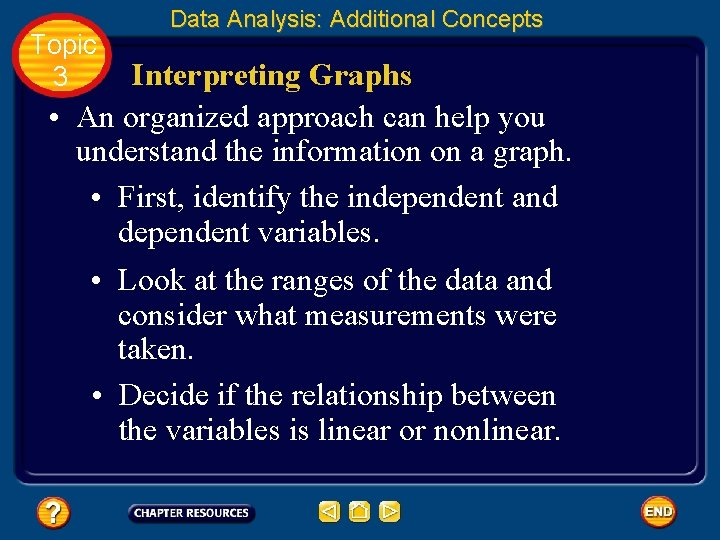Topic 3 Data Analysis: Additional Concepts Interpreting Graphs • An organized approach can help you understand the information on a graph. • First, identify the independent and dependent variables. • Look at the ranges of the data and consider what measurements were taken. • Decide if the relationship between the variables is linear or nonlinear.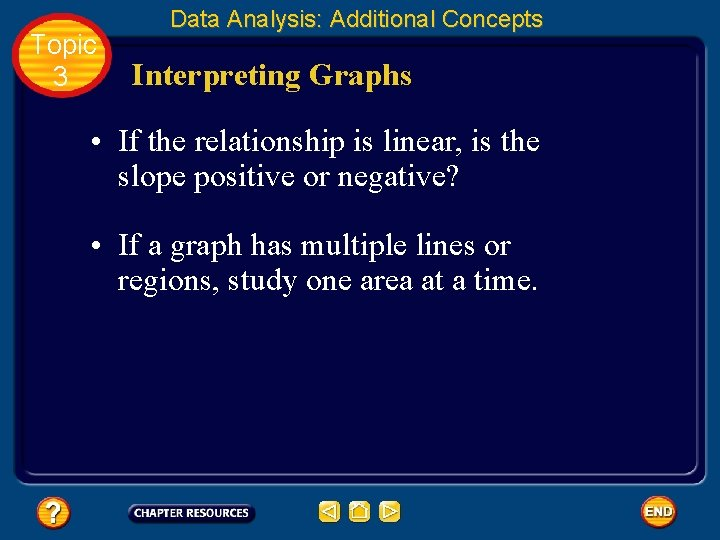Topic 3 Data Analysis: Additional Concepts Interpreting Graphs • If the relationship is linear, is the slope positive or negative? • If a graph has multiple lines or regions, study one area at a time.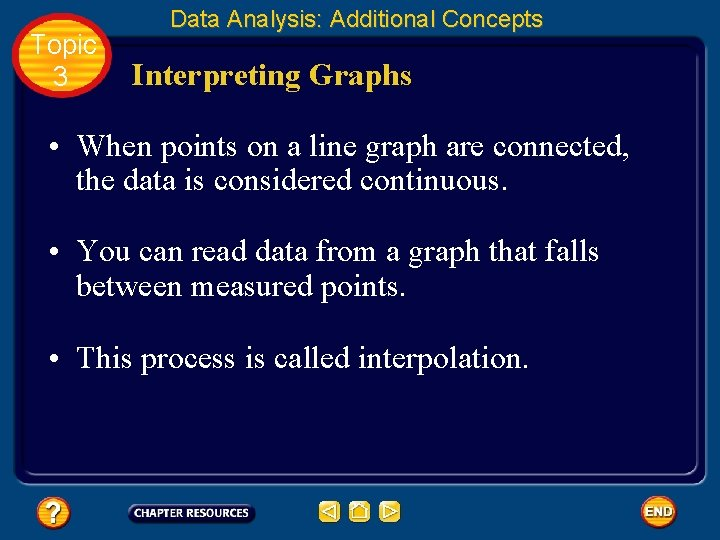Topic 3 Data Analysis: Additional Concepts Interpreting Graphs • When points on a line graph are connected, the data is considered continuous. • You can read data from a graph that falls between measured points. • This process is called interpolation.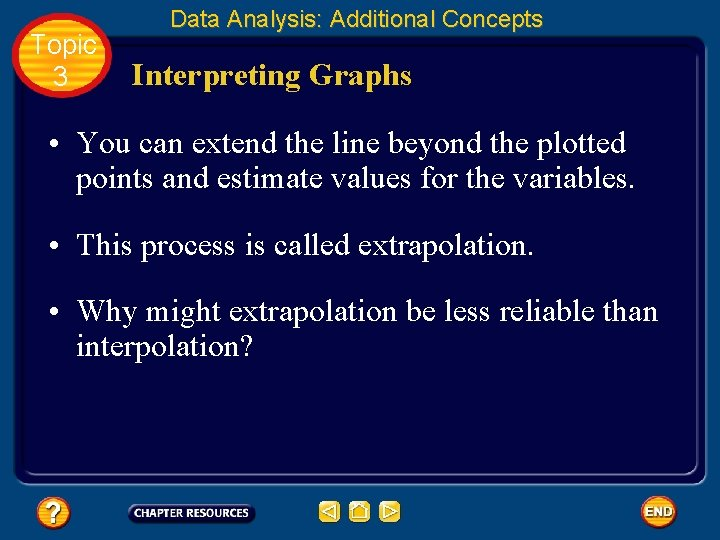Topic 3 Data Analysis: Additional Concepts Interpreting Graphs • You can extend the line beyond the plotted points and estimate values for the variables. • This process is called extrapolation. • Why might extrapolation be less reliable than interpolation?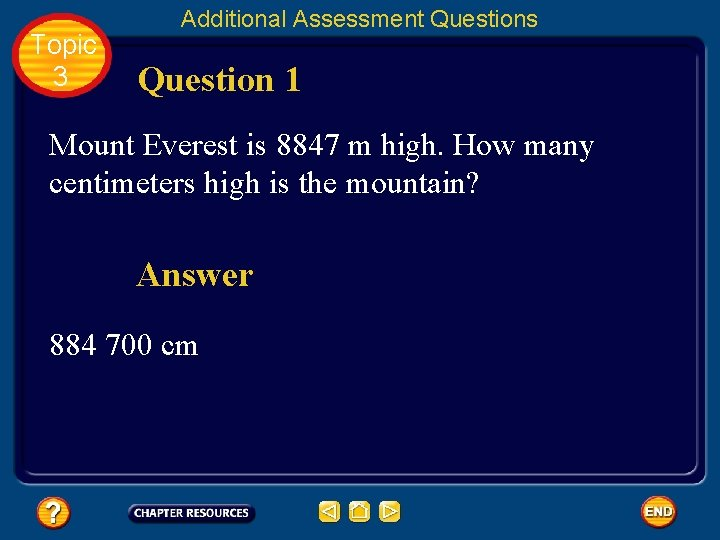Topic 3 Additional Assessment Questions Question 1 Mount Everest is 8847 m high. How many centimeters high is the mountain? Answer 884 700 cm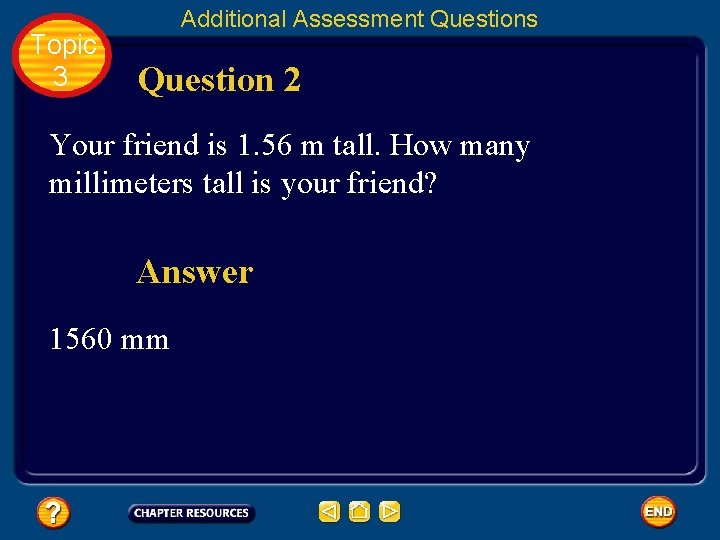Topic 3 Additional Assessment Questions Question 2 Your friend is 1. 56 m tall. How many millimeters tall is your friend? Answer 1560 mm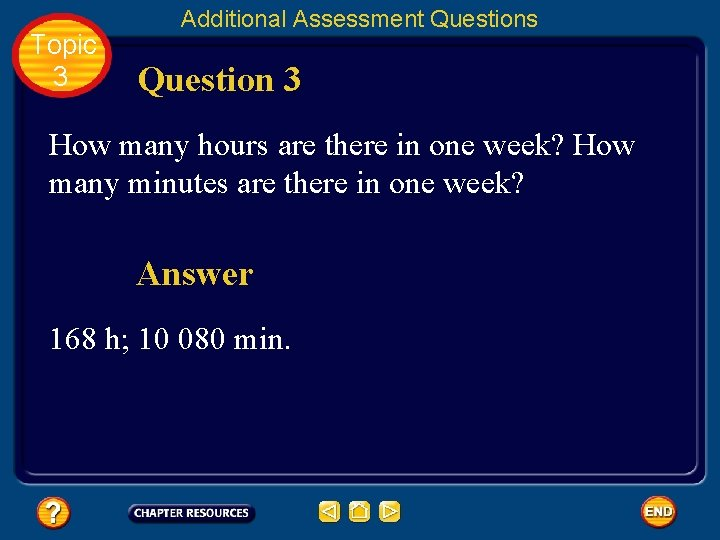Topic 3 Additional Assessment Questions Question 3 How many hours are there in one week? How many minutes are there in one week? Answer 168 h; 10 080 min.